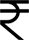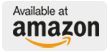## Introductory R for Beginners: A Beginners Guide To R

9789387445536
9788193445266
B.N. Mandal
276
2018
Hardbound1995This book is a complete beginner’s guide to using and learning R. It covers topics on downloading and installing R, using R functions, creating R functions and using R packages. The book discusses how to read data into R and then how to use R for various data manipulation purposes. Computing descriptive statistics and statistical test of hypotheses are also discussed at length using R.

1. Introduction
2. Functions in R
3. R packages